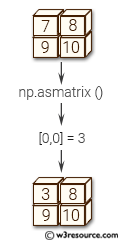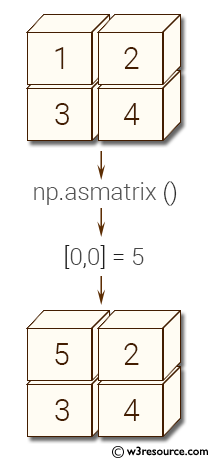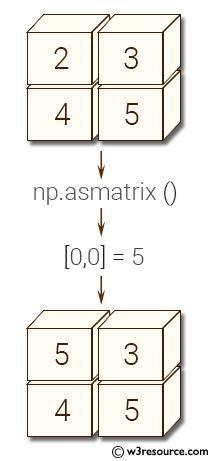# NumPy array creation: asmatrix() function

## asmatrix() function

The asmatrix() function is used to interpret the input as a matrix.
Unlike matrix, asmatrix does not make a copy if the input is already a matrix or an ndarray. Equivalent to matrix(data, copy=False).

Syntax:

`numpy.asmatrix(data, dtype=None)`Version: 1.15.0

Parameter:

Name Description Required /
Optional
data Input data. Required
dtype Data-type of the output matrix. optional

Return value:

mat : matrix
data interpreted as a matrix.

Example-1: NumPy.asmatrix() function

``````>>> import numpy as np
>>> x = np.array([[1,2], [3,4]])
>>> n = np.asmatrix(x)
>>> x[0,0] = 5
>>> n
matrix([[5, 2],
[3, 4]])
``````

Pictorial Presentation:Example-2: NumPy.asmatrix() function

``````>>> import numpy as np
>>> a = np.array([[2,3], [4,5]])
>>> x = np.asmatrix(a)
>>> a[0,0] = 5
>>> x
matrix([[5, 3],
[4, 5]])
``````

Pictorial Presentation:Python - NumPy Code Editor:

Previous: ascontiguousarray()
Next: copy()

﻿